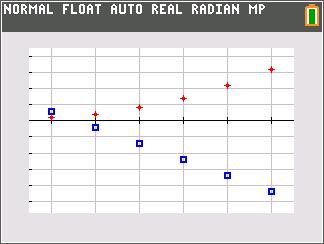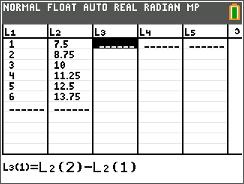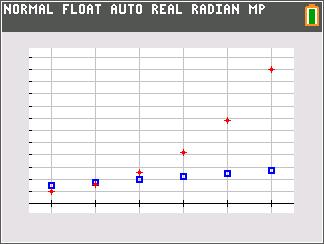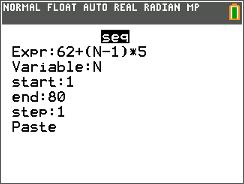### Mathematics lessons for IB® Diploma Programme

Analysis and Approaches | Statistics and Probability## Arithmetic Sequences and Series

### Activity Overview

Students use formulas to find the differences of the consecutive terms, plot a scatter plot of each sequence, and determine that sequences with common differences (called arithmetic sequences) have scatter plots whose points form a straight line.

## Key Steps## Step 1

In problem one, students will use formulas to find the differences of the consecutive terms of a sequence.## Step 2

Then, students will plot a scatter plot of each sequence and determine that sequences with common differences, also called arithmetic sequences, have scatter plots that form a straight line.## Step 3

In problems two and three, students generate a sequence and then find the sum of the first n terms of a sequence using a formula.